Search by Topic

Resources tagged with Graphs similar to N Is a Number:

Filter by: Content type:
Age range:
Challenge level:

There are 35 results

Broad Topics > Functions and Graphs > GraphsLap Times

Age 14 to 16 Challenge Level:

Can you find the lap times of the two cyclists travelling at constant speeds?Walk and Ride

Age 7 to 14 Challenge Level:

How far have these students walked by the time the teacher's car reaches them after their bus broke down?Age 14 to 16 Challenge Level:

Four vehicles travel along a road one afternoon. Can you make sense of the graphs showing their motion?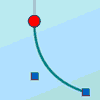Graphical Interpretation

Age 14 to 16 Challenge Level:

This set of resources for teachers offers interactive environments to support work on graphical interpretation at Key Stage 4.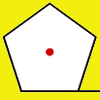How Far Does it Move?

Age 11 to 14 Challenge Level:

Experiment with the interactivity of "rolling" regular polygons, and explore how the different positions of the red dot affects the distance it travels at each stage.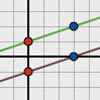Parallel Lines

Age 11 to 14 Challenge Level:

How does the position of the line affect the equation of the line? What can you say about the equations of parallel lines?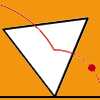Speeding Up, Slowing Down

Age 11 to 14 Challenge Level:

Experiment with the interactivity of "rolling" regular polygons, and explore how the different positions of the red dot affects its speed at each stage.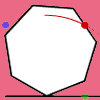Up and Across

Age 11 to 14 Challenge Level:

Experiment with the interactivity of "rolling" regular polygons, and explore how the different positions of the red dot affects its vertical and horizontal movement at each stage.Age 14 to 16 Challenge Level:

Four vehicles travelled on a road. What can you deduce from the times that they met?Spaces for Exploration

Age 11 to 14

Alf Coles writes about how he tries to create 'spaces for exploration' for the students in his classrooms.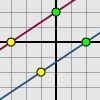Translating Lines

Age 11 to 14 Challenge Level:

Investigate what happens to the equation of different lines when you translate them. Try to predict what will happen. Explain your findings.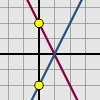Reflecting Lines

Age 11 to 14 Challenge Level:

Investigate what happens to the equations of different lines when you reflect them in one of the axes. Try to predict what will happen. Explain your findings.Which Is Cheaper?

Age 14 to 16 Challenge Level:

When I park my car in Mathstown, there are two car parks to choose from. Can you help me to decide which one to use?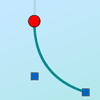Age 14 to 16 Challenge Level:

Can you adjust the curve so the bead drops with near constant vertical velocity?Which Is Bigger?

Age 14 to 16 Challenge Level:

Which is bigger, n+10 or 2n+3? Can you find a good method of answering similar questions?Motion Sensor

Age 14 to 16 Challenge Level:

Looking at the graph - when was the person moving fastest? Slowest?Matchless

Age 14 to 16 Challenge Level:

There is a particular value of x, and a value of y to go with it, which make all five expressions equal in value, can you find that x, y pair ?Bus Stop

Age 14 to 16 Challenge Level:

Two buses leave at the same time from two towns Shipton and Veston on the same long road, travelling towards each other. At each mile along the road are milestones. The buses' speeds are constant. . . .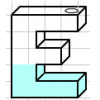Maths Filler

Age 11 to 14 Challenge Level:

Imagine different shaped vessels being filled. Can you work out what the graphs of the water level should look like?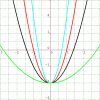Age 14 to 16 Challenge Level:

Here are some more quadratic functions to explore. How are their graphs related?What's That Graph?

Age 14 to 16 Challenge Level:

Can you work out which processes are represented by the graphs?Electric Kettle

Age 14 to 16 Challenge Level:

Explore the relationship between resistance and temperatureImmersion

Age 14 to 16 Challenge Level:

Various solids are lowered into a beaker of water. How does the water level rise in each case?Maths Filler 2

Age 14 to 16 Challenge Level:

Can you draw the height-time chart as this complicated vessel fills with water?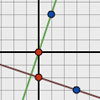Perpendicular Lines

Age 14 to 16 Challenge Level:

Position the lines so that they are perpendicular to each other. What can you say about the equations of perpendicular lines?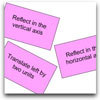Surprising Transformations

Age 14 to 16 Challenge Level:

I took the graph y=4x+7 and performed four transformations. Can you find the order in which I could have carried out the transformations?Bio Graphs

Age 14 to 16 Challenge Level:

What biological growth processes can you fit to these graphs?Fence It

Age 11 to 14 Challenge Level:

If you have only 40 metres of fencing available, what is the maximum area of land you can fence off?Diamond Collector

Age 11 to 14 Challenge Level:

Collect as many diamonds as you can by drawing three straight lines.Exploring Cubic Functions

Age 14 to 18 Challenge Level:

Quadratic graphs are very familiar, but what patterns can you explore with cubics?Parabolas Again

Age 14 to 18 Challenge Level:

Here is a pattern composed of the graphs of 14 parabolas. Can you find their equations?Ellipses

Age 14 to 18 Challenge Level:

Here is a pattern for you to experiment with using graph drawing software. Find the equations of the graphs in the pattern.More Parabolic Patterns

Age 14 to 18 Challenge Level:

The illustration shows the graphs of twelve functions. Three of them have equations y=x^2, x=y^2 and x=-y^2+2. Find the equations of all the other graphs.Parabolic Patterns

Age 14 to 18 Challenge Level:

The illustration shows the graphs of fifteen functions. Two of them have equations y=x^2 and y=-(x-4)^2. Find the equations of all the other graphs.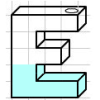Mathsjam Jars

Age 14 to 16 Challenge Level:

Imagine different shaped vessels being filled. Can you work out what the graphs of the water level should look like?